Working with Sequences (in both Seq and Func Modes)
 In Seq MODE:

To work with sequences in the Seq mode:

• Press MODE key

• Choose Seq in the fourth line

• Hit ENTER to highlight Seq

• Leave other settings as default (on the left side)Press Y= and notice the difference in this screen.

To define a sequence, you must specify:

• nMin -  where you will start counting the numbers
in the sequence (most likely this value is 1)
• u(n) - the pattern for the sequence
(use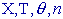key to enter the pattern)
• u(nMin) - the first number in the sequence
(When you enter the first number in your sequence, notice the list braces that appear.  These braces will allow for the entry of more than one term if needed.)Example 1:

Consider the sequence defined as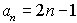.
List the first 6 terms of the sequence and find the 105th term.

 Enter the information in Y=:Set the table (TBLSET):Look at the table (TABLE):The first 6 terms are easily visible in the table.

To find the 105th term, reset the TblStart to a value near (or at)  the desired term.If you GRAPH when in sequence mode, even if your calculator is set in connected mode, you will only see plots of points.   Remember that a sequence is a listing of numbers without other values in between.  Notice, in the center calculator screen below, how the sequence mode table shows answers only for n values of 1 or larger.  Remember that the domain of a sequence is the set of natural numbers
{1, 2, 3, ...}.If you express this sequence as a function and return to function mode, you will see a connected graph (a line in this case), rather than the plotted points.

Example 2:  (Recursive sequence)   List the first 7 terms of the sequence: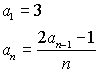Enter the recursive formula information. Enter the u by using the alpha letter u (2nd 7).  Note the starting value of 3.View the terms of the sequence in the table.Example 3:  Generate the Fibonacci Sequence (where each term, after the first two terms, is determined by adding the two previous terms).

 Enter the pattern for the sequence. Notice how the u(nMin) displays the first two terms using the list braces.Go to the table to view the Fibonacci sequence.In Func MODE:
 Certain sequences can be examined in Func mode.  Consider the following examples: Example 4:  Arithmetic and geometric sequences can be quickly generated in Func mode if the common difference or common ratio, respectively, are known, along with the starting value.List the first five terms of an arithmetic sequence whose first term is 1 and whose common difference is 4.

 A "quick - see it on the screen" method: Enter the first term on the home screen. Press ENTER to register the first term. Press + 4 (the expression Ans+4 will appear). Press ENTER. Continuing to press ENTER will generate successive terms in this sequence.Unfortunately, finding specific terms with this method, such as the 100th, will require that you press ENTER and count very carefully - not always an easy process.

Example 5:  If an expression/formula is known for the generation of a sequence, the terms can be listed in Func mode using the seq( function.

List the first 6 terms of the sequence defined by: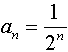2nd STAT (LIST) → OPS Choose #5 seq(Using seq(, type the formula, variable, starting value for n, last value of n needed, increment.  (For ease, X may be used as the variable -- or type N using the alpha key.)  Arrow to the right to see more of the terms of the sequence. If you need fractional answers, utilize the MATH - #1►FracThis method of listing terms of a sequence will be limited to 999 terms of the sequence at any one time.

Example 6:

If an expression/formula is known for the generation of a sequence, the sum of a specific number of terms can be found in Func mode.

Find the sum of the first 10 terms of the sequence defined by: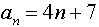2nd STAT (LIST) → MATH Choose #5 sum(Notice that sum is followed by the entry of the sequence as shown in Example 5.Find the sum of the second through fifth terms of the sequence:Example 7:

Sequences represented by a recursive formula can be generated in Func mode.

List the first 4 terms of the sequence defined by: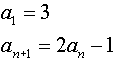Type the starting value and press ENTER. Use the ANS function to create the formula used to generate the sequence.  Type 2*ANS-5 and press ENTER. Now, simply press ENTER to create successive terms of the sequence.